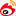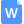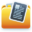帮帮文库欢迎您！提示：若您对当前内容不满意，强烈建议在右侧相似文档里加载系统推荐的类似文档，您也可以在上方搜索框输入关键词进行随意搜索！

#（独家原创）MQ100_门式起重机总体计算书设计（全套CAD图纸完整版）

✔ 可以下载到电脑 ✔ 可随意编辑修改 ✔ 支持电脑打印 ✔ 下载无本网站水印本资源为压缩包，下载后将获得以下所有文档， dwg 格式为CAD图纸，展示的仅是截图，下载后图纸原稿无水印可编辑。（图纸） MQ100.00.dwg（其他） MQ100总体计算书.doc（其他） 说明.htm1 点击【下载】按钮，注册或登陆账号 2 登陆后再点【下载】按钮，在弹出的窗口选择下载方式，扫码付款
3 付款后关掉下载提示，重新点【下载】按钮 4 在弹出的窗口，点【下载文档到电脑】，便可以在电脑打开编辑了相似文档（阅读对比找最满意）

2、gm回转惯性力到轨道上平面的力矩：M==kgm()行走惯性力F=(＋＋＋)=kg行走惯性扭矩：T==kgm行走惯性力到轨道上平面的力矩：M==kgm(四)风载荷A、工作，垂直风（风向与臂架垂直）臂长jib=m，垂直风名称迎风面积(m)回转半径(m)工作风力(kg)风扭矩(k。回转、行走、风AB重力:kg重力力矩:kgm回转力矩:kgm行走力矩:kgm风力矩:kgmR=(－)＋(＋)()＋()=－kgR=(－)－()－()=－kgR=(－)－(＋)()－()=－kgR=(－)＋()＋()=－kg工况：非工作、前吹风（R=m，Q=）DC风AB重力:kg重力力矩:－kgm风力矩:kgmR=－＋()＋()=＋kgR为正，故按三点支承计算R=－()－()=－kgR=R=－－()－()=－。

3、机构起重臂端部节起重臂拉杆平衡臂结构平衡臂拉杆配kgm风力矩:kgmR=(－)＋(＋)()＋()=－kgR=(－)－()－()=－kgR=(－)－(＋)()－()=－kgR=(－)＋()＋()=－kg工况：非工作、前吹风（R=m，Q=）DC风AB重力:kg重力力矩:－kg。

4、门式起重机设计计算完全按《起重机设计规范》GB执行,并参照下列标准进行设计计算:《塔式起重机设计规范》GBT《法国塔式起重机设计规范》NFE工作级别A利用等级U起升机构M变幅机构M回转机构M行走机构M最大幅度m最大起重量Kg（一）基本参数:回转速度rmin回转制动时间s行走。边M=(＋)=kgmM=(－)=kgmMM==gt四、M行走式门式起重机的台车支反力计算工况：工作、后吹风（R=m，Q=t）DC回转、行走、风AB重力:kg重力力矩:kgm回转力矩:kgm行走力矩:（－）＋=kgmMM==gt工况：工作、动态、有风（R=m，Q=t）回转、行走、后吹风M=M＋M＋M=（－）＋＋=kgmMM==gt工况：工作、动态、突然卸载（R=m，Q=t）无回转、无行走、风M=M＋M=（＋）。

5、）m倾翻边M=(＋)=kgmM=(－)=kgmMM==gt四、M行走式门式起重机的台车支反力计算工况：工作、后吹风（R=m，Q=t）DC回转、行走、风AB重力:kg重力力矩:kgm回转力矩:kgm行走力矩:kgm风力矩:kgmR=(－)＋(＋)()＋()=－kgR=(－)。

6、m)标高(m)起重臂根部节m小车牵引机构起重臂端部节起重臂拉杆平衡臂结构平衡臂拉杆配重起升机构(含钢丝绳)塔头称重滑轮驾驶室(含电气系统)回转支MQ+门式起重机总体计算书设计ltgtMQ门式起重机总体设计计算书(共页，含封面)XXX机械工程研究所年月一总体计算计算原则:MQ。